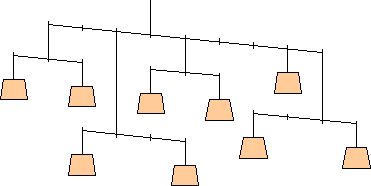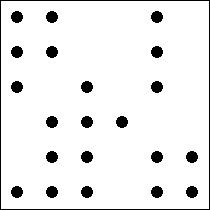2008 U. S. Championship Puzzles

1. Tilted Weights

Assign the values 1 to 9 to the weights in the diagram so that each balance is tilted one unit to the right (that is, at each fulcrum, the total torque on the right side is one unit greater than on the left).2. Dot Triangles

Determine the seven triangles from the 21 points given. Each triangle is a right triangle with one leg twice as long as the other. Each point is used as a vertex by exactly one triangle, although triangles may overlap.